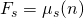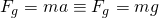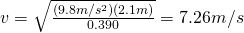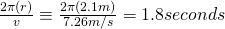# Minimum velocity related to coefficent of friction

A question posed in Physics class (much help to Judy for the assistance on this one):

One of the rides found at carnivals is the rotating cylinder. The riders step inside the vertical cylinder and stand with their backs against the curved wall. The cylinder spins very rapidly, and at some angular velocity, the floor is pulled away. The thrill-seekers now hang like flies on the wall. If the radius of the cylinder is 2.1 m and the coefficient of static friction between the people and the wall is ms = 0.390, what is the maximum period of rotation (in s) of the cylinder for the floor to be removed safely?

Now, what’s important to note out of all this is that you’re looking to get the angular velocity that will counter the force of gravity enough that static friction is effective in holding the people against the wall.

We know:

Coefficient of static friction = 0.390
Gravitational Acceleration = 9.8 m/s^2

We also know that static friction must be greater than or equal to the force and that must be greater than or equal to 0 in order to remain in place.  Anything less than 0 and the object moves.  So, we need 2 equations for this, one for the force related to gravity and one related to the normal force related to friction:Now, the key to this is realizing that the normal force is related, or rather, equal to, the amount of force needed to keep the person in place.  The formula for that is:These can be combined as follows:Now you can plug in numbers for the variables and get an answer for velocity.Now the second key to this is remembering that the distance around a circle is its circumference, that is the value that you’ll need to put in to get your velocity value spitting out seconds around.And there you have it.  The minimum velocity that the cylinder needs to be spinning is 7.26 m/s which will cause it to rotate once every 1.8 seconds keeping the riders firmly in place.

As always, please correct me if you find any errors.